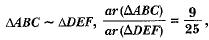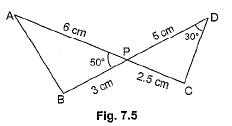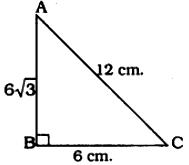Courses

# Practice Test: Triangles

## 10 Questions MCQ Test Mathematics (Maths) Class 10 | Practice Test: Triangles

Description
This mock test of Practice Test: Triangles for Class 10 helps you for every Class 10 entrance exam. This contains 10 Multiple Choice Questions for Class 10 Practice Test: Triangles (mcq) to study with solutions a complete question bank. The solved questions answers in this Practice Test: Triangles quiz give you a good mix of easy questions and tough questions. Class 10 students definitely take this Practice Test: Triangles exercise for a better result in the exam. You can find other Practice Test: Triangles extra questions, long questions & short questions for Class 10 on EduRev as well by searching above.
QUESTION: 1

Solution:
QUESTION: 2

### Sides of triangles are (i) 3 cm, 4 cm, 6 cm. (ii) 4 cm, 5 cm, 6 cm. (iii) 7 cm, 24 cm, 25 cm (iv) 5 cm, 12 cm, 14 cm. Which of these is right triangle?

Solution:

On verification, triangle with sides 7 cm, 24 cm and 25 cm is a right triangle
∵ (25)2 = (7)2 + (24)2.

QUESTION: 3

### IfBC = 21 cm, then EF is equal to

Solution:
QUESTION: 4

A square and a rhombus are always

Solution:
QUESTION: 5

It is given that ΔABC ~ ΔDFE, ∠A = 30°, ∠C = 50°, AB = 5 cm, AC = 8 cm and DF = 7.5 cm. Then, the following is true:

Solution:
QUESTION: 6

In Fig. 7.5, two line segments AC and BD intersect each other at the point P such that PA = 6 cm, PB = 3 cm, PC = 2.5 cm, PD = 5 cm, ∠APB = 50° and ∠CDP = 30°. Then, ∠PBA is equal toSolution:
QUESTION: 7

In ΔLMN and ΔPQR, ∠L = ∠P, ∠N = ∠R and MN = 2QR. Then the two triangles are

Solution:
QUESTION: 8

If in triangles ABC and DEF, AB/EF = AC/DE, then they will be similar when

Solution:
QUESTION: 9

It is given that ar(ΔABC) = 81 square units and ar(ΔDEF) = 64 square units. If ΔABC ~ ΔDEF, then

Solution:
QUESTION: 10

In triangle ABC, if AB = 6√3 cm, AC = 12 cm and BC = 6 cm, then ∠B is

Solution:Here largest side is 12 cm.
If the square of the hypotenuse is equal to the square of the other two sides, then it is a right-angled triangle.

∴ c2 = a2 + b2

AC2 = AB2 + BC2

(12)2 = (63)2 + (6)2

44 = 36 × 3 + 36

144 = 108 + 36

144 = 144

∴ ∆ABC is a right angled triangle and angle opposite to hypotenuse, ∠B = 90°.